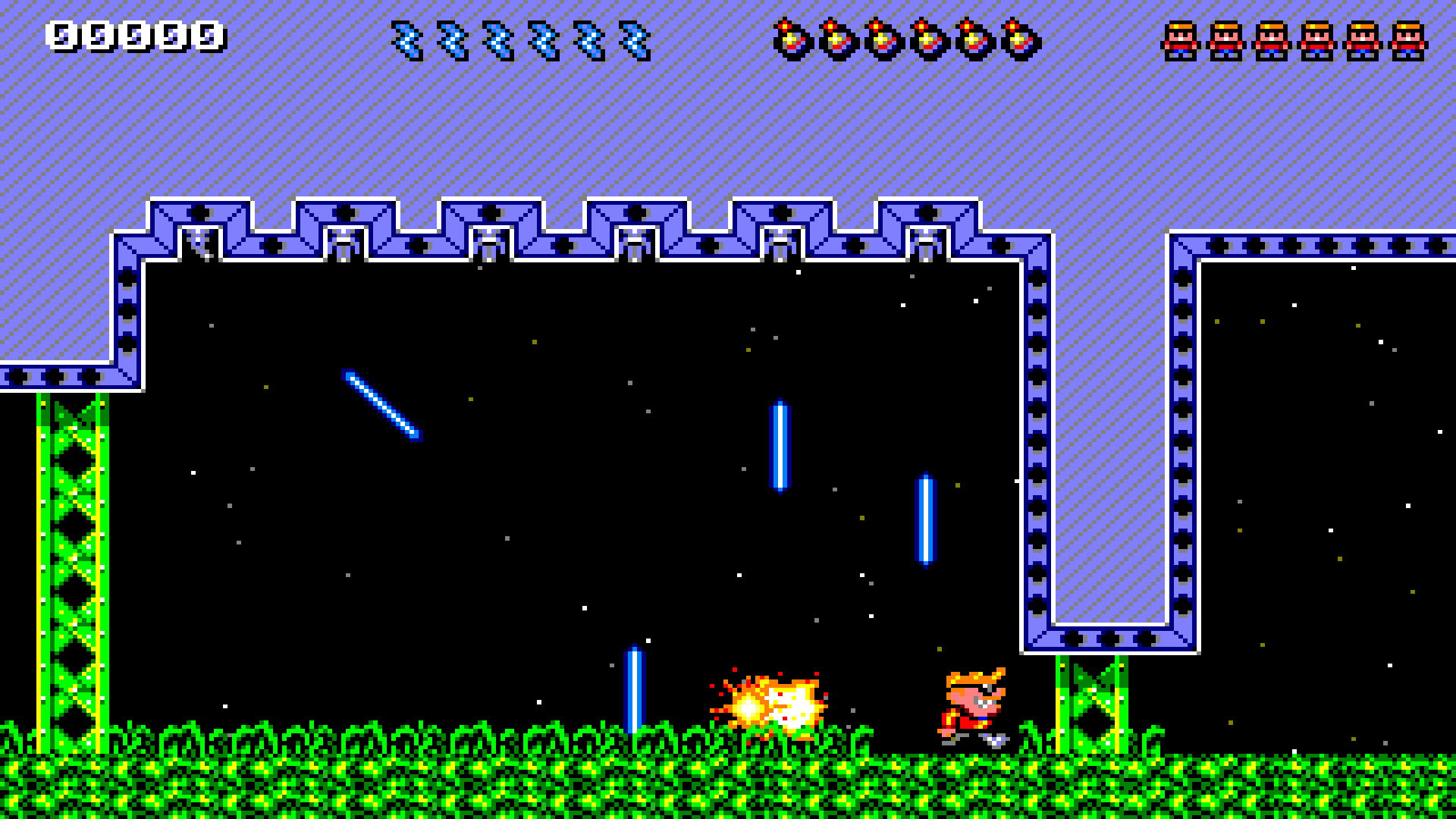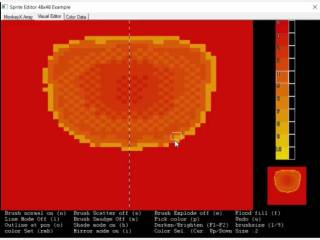-=+=- -=+=- -=+=- -=+=- -=+=- -=+=- -=+=- -=+=- -=+=- -=+=- -=+=- -=+=- -=+=- -=+=- -=+=- -=+=- -=+=- -=+=- -=+=- -=+=- -=+=- -=+=- -=+=- -=+=- -=+=- -=+=- -=+=- -=+=- -=+=- -=+=- (c) WidthPadding Industries 1987 0|500|0 -=+=- -=+=- -=+=- -=+=- -=+=- -=+=- -=+=- -=+=- -=+=- -=+=- -=+=- -=+=- -=+=- -=+=- -=+=- -=+=- -=+=- -=+=- -=+=- -=+=- -=+=- -=+=- -=+=- -=+=- -=+=- -=+=- -=+=- -=+=- -=+=- -=+=-

### Welcome to Socoder

Socoder is a community of coders, who sit in a quiet corner of the internet, chatting endlessly about random nonsense.
Occasionally we code, too.

If you'd like to join in with the chatter, feel free to register.

# Navigation Tips

On the top left is a little cog which you can use to open the Settings sidebar, where you'll find the registration and login areas. Once you're logged in, this is also where most of the menu-based things will appear.
On the top right is a secondary sidebar button which can be used to reveal the most recent topics. You may dock either of these sidebars into place, using the provided links.
As well as these, there's a paintbrush on the top right to help you style the site using a variety of themes and other such settings.

# Cookie Information

therevillsgames# Jack Deadly

4th April 2019When Rick dies, Jack steps up!

Jayenkai# JNKPlat 2018

2nd October 2018Platdude's stuck in the dungeon again!

Pakz# Blitz Basic Sprite Editor

24th August 2018Sprite Editor

Jayenkai# JNKTitler

24th August 2018Create Titlecards semi-randomly

TomToad# Marble Maze (itch.io #lowrezjam2018)

12th August 2018Here is my submission for the #lowrezjam2018 jam,
Marble Maze

Edit to add: Used BlitzMax-NG, Pyxel Edit, and TileD to create this.

Jayenkai# Generic Snake Clone

29th July 2018Go, Snake! Go!!